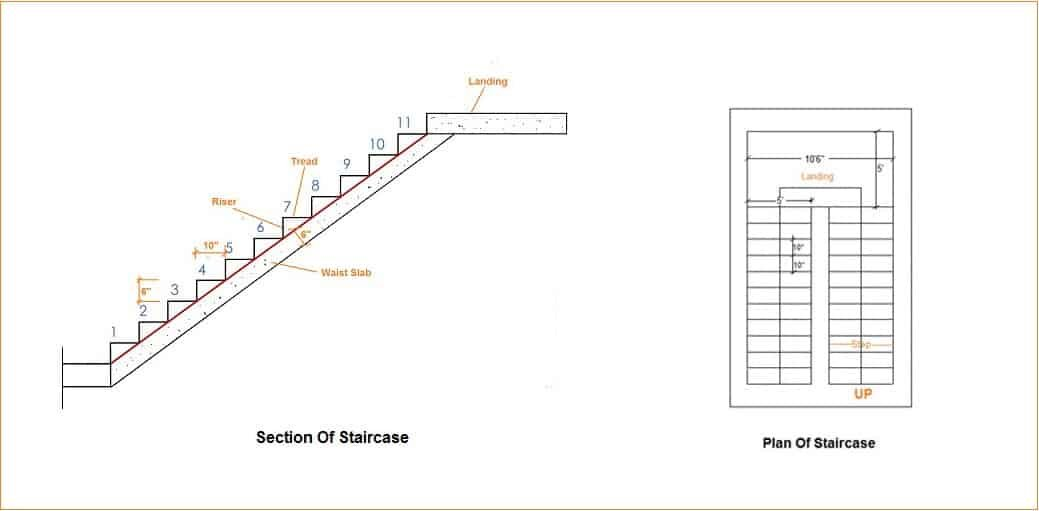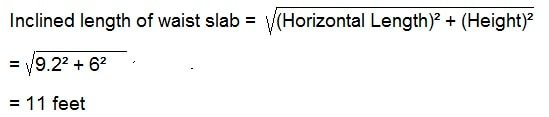# How To Calculate Volume Of Concrete For Staircase

Contents

## How To Calculate Volume Of Concrete For Staircase:

The staircase is one of the essential parts of a building. It helps people to access different floors in a building. In this article, I will explain how to estimate concrete volume and quantity of cement, sand, aggregates for a staircase. Before starting the estimation, we need to know different components of a staircase which I will use in this calculation.

## Components Of Staircase:

### Steps:

A portion of stairway comprising the tread and riser which permits ascent and descent from one floor to another.

### Tread:

The horizontal upper part of a step on which foot is placed in ascending or descending stairway.

### Riser:

The vertical portion of a step providing support to the tread.

### Flight:

A series of steps without any platform, break, or landing in their direction.

### Landing:

A platform or resting place provided between two flights.

Now coming to the calculation of concrete volume for the staircase.

Let’s take an example:The height of one floor = 12 feet.

Height to be acquired by one flight = 12/2 = 6 feet.

Risers = 6″ = 0.5 feet.

Number of risers = Height of flight / Riser = 6/0.5 = 12

Tread = 10″ = 0.8 feet.

Number of treads = (Numbers of risers -1) = 12 – 1

Thickness of waist slab = 6″ = 0.5 feet

Length of step = 5 feet

### Volume Of Concrete For Steps:

Volume of one step = 1/2 x riser x tread x length of step

= 1/2 x 0.5 x 0.8 x 5 = 1.03 = 1 cubic feet.

As we have 11 steps in a flight the volume of steps for the first flight  = 11 x 1 = 11 cubic feet.

### Concrete Volume For Waist Slab:

To calculate the volume of concrete for the waist slab we need to know the inclined length of the waist slab.

The horizontal length of waist slab = Tread x Number of steps

=0.8333′ x 11 = 9.2 feet.

The height of the top of the landing from floor = Number of riser x Height of riser

= 12 x 0.5 = 6 feet.The volume of concrete for waist slab = Inclined length of waist slab x Width of waist slab x Thickness of waist slab

= 11′ x 5′ x 0.5′

= 27.5 cubic feet

∴ The volume of concrete for first flight = Volume of waist slab + Volume of steps

= 27.5+11 = 38.5 cubic feet

### Volume Of Concrete For Landing Of Staircase:

Length of landing = 10.5 feet

Width of landing = 5 feet

The thickness of landing = 0.5 feet

∴ Concrete volume for landing = 10.5′ x 5′ x 0.5′ =  26 cubic feet.

### Concrete Volume for 2nd Flight Of The Staircase:

As the 1st light and 2nd flight are the same in our staircase so the volume of concrete will be the same.

Concrete volume for 2nd flight of the staircase = 38.5 cubic feet.

Total volume of concrete for staircase = Volume of first flight + Volume of second flight + Volume of landing

= 38.5 + 38.5 + 26

= 103 cubic feet.

Well, this is the wet volume of concrete. To convert wet volume into dry volume we need to multiply with 1.54

Dry volume of concrete = 103 x 1.54 = 159 cubic feet.

### Quantity Of Materials For Staircase:

We will calculate for 1:2:4 Concrete mix.

1. Volume of cement = 1/7 x Dry volume = 1/7 x 159 = 22.66 cubic feet.

Number of Cement bags = volume of cement / Volume of cement bag  = 22.66 / 1.25 = 18 No’s of bag. (The volume of one bag cement of 50 kg = 1.25 cubic feet)

2. Volume of Sand = 2/7 x Dry volume = 2/7 x 159 = 45 cubic feet.

3. Volume of Aggregate = 4/7 x Dry volume = 4/7 x 159 = 91 cubic feet.

Note: Here I have used cubic feet unit and 1:2:4 mix ratio, you can use cubic meter unit and any other mix ratio of concrete.

### 5 thoughts on “How To Calculate Volume Of Concrete For Staircase”

1. Meaning of the triangular box in d calculation for volume of concrete in staircase diagram above

Reply
2. Good morning sir, How do you get the length of the step to be 5ft. Is it the Length of 1 step? Because you use it to calculate the volume of concrete for one step and then you later multiply the result by 11(no of steps) to get the volume of concrete for the steps. Please Check the calculations. Kind regards

Reply
• Hey see the plan of the staircase, there we assumed length of each step as 5 ft. Thanks

Reply
3. weldone
sir i umferstood it easily thnks sir

Reply
4. Hindi me send kiya kare

Reply
error: Content is protected !!